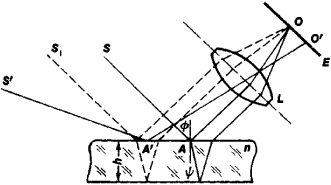Fringes of Equal Inclination

The following article is from The Great Soviet Encyclopedia (1979). It might be outdated or ideologically biased.

Fringes of Equal Inclination

a pattern of alternating light and dark bands observed when a transparent layer of constant thickness (a plane-parallel plate) is illuminated with a diverging or converging beam of monochromatic light or with a nonparallel beam of rays of a more complex structure. Each fringe passes through those points of the layer on which the light rays are incident at the same angle φ—hence the term “fringes of equal inclination.” Such fringes are often included among the effects of the optics of thin films, although they also arise in plates of comparatively large thickness.Figure 1. Fringes of equal inclination are formed on the screen E or on a photosensitive layer as a result of the collection by the lens L of parallel rays reflected from a plane-parallel plate. All rays incident on the plate in the plane of the figure at an angle θ—for example, the pairs of rays formed when rays S and S1 are split—are collected at one point (O) on the screen. Rays incident at a different angle will intersect in the focal plane of the lens on the screen at a different point (O′); only one such ray is illustrated here (S′).

The fringes appear as a result of interference between the light reflected from the front and rear boundaries of the plate (fringes of equal inclination in reflected light) or between the light transmitted through the plate without reflection and the light twice reflected by the surfaces of the plate (fringes of equal inclination in transmitted light). If the reflection coefficients r of the boundaries of the layer or plate are large, then the fringes may be well defined. Interference is possible because of the coherence of the rays, which follow different paths and consequently acquire a path difference. As a result of interference, a maximum or a minimum intensity in the reflected light—a light or dark fringe, respectively—will be observed (Figure 1) if the path difference between the two coherent beams is respectively equal to an integral number of wavelengths, that is,

2nh cos ψ + λ/2 = kλ/2

where n is the refractive index of the plate, h the plate’s thickness, λ the wavelength of the light, ψ the angle of refraction of the rays, and k an integer whose even values correspond to the maxima and whose odd values correspond to the minima of the light intensity. The additional term λ/2 in the expression for the path difference takes into consideration the phase shift upon reflection from the optically denser medium. Since the angle of refraction ψ is uniquely determined by the angle of incidence φ, all rays with the same φ acquire the same path difference. Thus, interference maxima and minima arise in the directions of equal inclination of the reflected rays.

Since the rays that acquire the same path differences—for example, the rays that arise when the rays S and S1 are split—move away from the plate parallel to each other, the fringes of equal inclination formed when these rays “intersect” are localized at infinity. In order to observe them, the interfering rays must be collected on a screen or photographic plate by means of a lens, or the eye must be accommodated to infinity. Fringes of equal inclination may be observed when the light source is extended by any amount. For converging or diverging illuminating beams, the fringes of equal inclination in the focal plane of a collecting lens L are circles or ellipses.

A change Δλ in the wavelength of the incident light causes a displacement of the fringes that is readily observed when h and r are large. Extensive use is made of this fact in spectral investigations employing Fabry-Perot, Jamin, and other interferometers. In spectral instruments of this type the fringes are used to study the complex structure of spectral lines. In order to observe the fringes at large h, it is necessary first to isolate a small spectral interval from the irradiating light—that is, to partially monochromatize the light; otherwise the fringes for different λ are superposed on each other and the interference pattern becomes unobservable.

Fringes of equal inclination are also used for highly precise monitoring of the quality of plane-parallel transparent plates, especially glass plates.

REFERENCES

Landsberg, G. S. Optika, 4th ed. Moscow, 1957. (Obshchii kurs fiziki, vol. 3.)
Kaliteevskii, N. I. Volnovaia optika. Moscow, 1971.
Born, M., and E. Wolf. Osnovy optiki, 2nd ed. Moscow, 1973. (Translated from English.)
Prosvetlenie optiki Edited by I. V. Grebenshchikov. Moscow-Leningrad, 1946.
Shishlovskii, A. A. Prikladnaia fizicheskaia optika. Moscow, 1961.

L. N. KAPORSKII